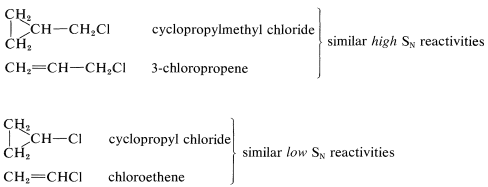# 14.6: Cycloalkyl Halides

$$\newcommand{\vecs}{\overset { \rightharpoonup} {\mathbf{#1}} }$$ $$\newcommand{\vecd}{\overset{-\!-\!\rightharpoonup}{\vphantom{a}\smash {#1}}}$$$$\newcommand{\id}{\mathrm{id}}$$ $$\newcommand{\Span}{\mathrm{span}}$$ $$\newcommand{\kernel}{\mathrm{null}\,}$$ $$\newcommand{\range}{\mathrm{range}\,}$$ $$\newcommand{\RealPart}{\mathrm{Re}}$$ $$\newcommand{\ImaginaryPart}{\mathrm{Im}}$$ $$\newcommand{\Argument}{\mathrm{Arg}}$$ $$\newcommand{\norm}{\| #1 \|}$$ $$\newcommand{\inner}{\langle #1, #2 \rangle}$$ $$\newcommand{\Span}{\mathrm{span}}$$ $$\newcommand{\id}{\mathrm{id}}$$ $$\newcommand{\Span}{\mathrm{span}}$$ $$\newcommand{\kernel}{\mathrm{null}\,}$$ $$\newcommand{\range}{\mathrm{range}\,}$$ $$\newcommand{\RealPart}{\mathrm{Re}}$$ $$\newcommand{\ImaginaryPart}{\mathrm{Im}}$$ $$\newcommand{\Argument}{\mathrm{Arg}}$$ $$\newcommand{\norm}{\| #1 \|}$$ $$\newcommand{\inner}{\langle #1, #2 \rangle}$$ $$\newcommand{\Span}{\mathrm{span}}$$

The cycloalkyl halides, except for cyclopropyl halides, have physical and chemical properties that are similar to those of the open-chain secondary halides and can be prepared by the same types of reactions (Table 14-5). All the cycloalkyl halides undergo $$S_\text{N}2$$ reactions rather slowly and, with nucleophiles that are reasonably basic ($$^\ominus \ce{OH}$$, $$^\ominus \ce{OC_2H_5}$$, $$^\ominus \ce{C \equiv N}$$, etc.), $$E2$$ reactions can be expected to predominate (Table 14-6). The rate of carbocation formation leading to $$S_\text{N}1$$ and $$E1$$ reactions is sensitive to ring size but, except for the small-ring halides, the carbocation reactions are normal in most other respects.

The cyclopropyl halides are exceptional in that their behavior is much more like alkenyl halides than like secondary alkyl halides. Thus cyclopropyl chloride undergoes $$S_\text{N}1$$ and $$S_\text{N}2$$ reactions much less rapidly than isopropyl or cyclohexyl chlorides. A relationship between the reactivity of cyclopropyl chloride and chloroethene is not surprising in view of the general similarity between cyclopropane rings and double bonds (Section 12-5). This similarity extends to cyclopropylmethyl derivatives as well. Cyclopropylmethyl chloride is reactive in both $$S_\text{N}1$$ and $$S_\text{N}2$$ reactions in much the same way as 3-chloropropene: